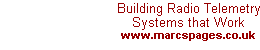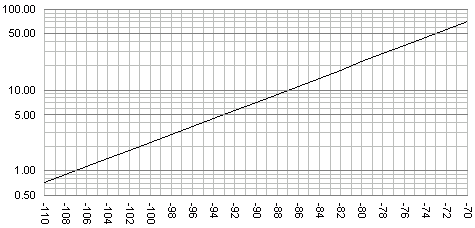Converting µV & dBm   Signal levels are often quoted in dBm (dB referenced to 1mW). To some radio engineers and technicians this is a meaningless figure, especially when it comes to SINAD measurement (in µV for 12dB SINAD). To this end the following graph was created to assist in converting dBm to µV at 50ohms (or vice versa).Although the graph only covers the range -110 to -70dBm (typical of scratchy to solid comms) any voltage can be calculated by applying the '20dB per decimal point' rule. +20dB moves the decimal point 'one to the right' (multiply by 10) and -20dB moves it 'one to the left' (divide by 10). An example would be a signal of 0.3µV would be -117.5dB, read by using the value for 3µV (-97.5) and adding -20dB to that to move the decimal point one place to the left. Useful figures. 0dBm = 1mW (regardless of impedance) 0dBm = 0.2236V @ 50ohms -73.01dBm = 50µV @ 50ohms (known as 'S9') -97.5dBm = 3µV @ 50ohms (generally accepted as a 'good' signal) | | Ask a Question | © 14.08.00